Courses

# Synchronous Machine of Electrical Machines

## 10 Questions MCQ Test GATE Electrical Engineering (EE) 2022 Mock Test Series | Synchronous Machine of Electrical Machines

Description
This mock test of Synchronous Machine of Electrical Machines for Electrical Engineering (EE) helps you for every Electrical Engineering (EE) entrance exam. This contains 10 Multiple Choice Questions for Electrical Engineering (EE) Synchronous Machine of Electrical Machines (mcq) to study with solutions a complete question bank. The solved questions answers in this Synchronous Machine of Electrical Machines quiz give you a good mix of easy questions and tough questions. Electrical Engineering (EE) students definitely take this Synchronous Machine of Electrical Machines exercise for a better result in the exam. You can find other Synchronous Machine of Electrical Machines extra questions, long questions & short questions for Electrical Engineering (EE) on EduRev as well by searching above.
QUESTION: 1

Solution:
QUESTION: 2

### The phasor diagram of a synchronous machine connected to an infinite bus is shown below. The machine is acting as a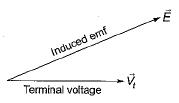Solution:

As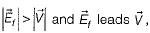therefore machine is acting as a generator and operating at lagging Pf.

QUESTION: 3

### A 6-pole alternator with 36 slots carries a 3-phase distributed winding. Each coil is short-pitched by one slot. The winding factor is given by

Solution:

Number of slots/poIe/phase,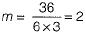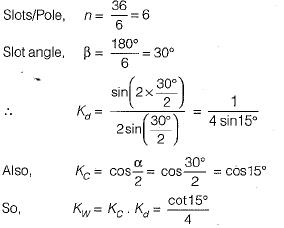QUESTION: 4

A winding is distributed in the slots along the air-gap periphery
1. to add mechanical strength to the winding.
2. to reduce the amount of conductor material required.
3. to reduce the harmonics in the generated emf.
4. to reduce the size of the machine.
5. for full utilization of iron and conductor materials.
From these, the correct answer is

Solution:
QUESTION: 5

Which of the following graphs represents the speed-torque characteristic of a synchronous motor?

Solution:

For a synchronous motor, N = Ns for all value of torque.

QUESTION: 6

In figure the characteristic that corresponds to the variation of synchronous reactances of synchronous machine with the field current is​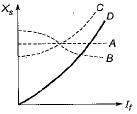Solution:
QUESTION: 7

The four methods of calculating voltage regulation of a 3-phase alternator are:
1. Emf method
2. Saturated synchronous reactance method
3. New ASA method
4. Mmf method
The correct sequence in the ascending order of the values of regulation obtained by these methods is

Solution:
QUESTION: 8

The flux per pole in a synchronous motor with the field circuit ON and the stator disconnected from the supply is found to be 25 mWb. When the stator is connected to the rated supply with the field excitation unchanged, the flux per pole in the machine is found to be 20 mWb while the motor is running on no-load. Assuming no-load losses to be zero, the no-load current drawn by the motor from the supply

Solution:

Since after connecting the stator to supply, flux per pole is reduced to 20 mWb from initial value of 25 mWb, therefore effect of armature reaction is demagnetizing i.e. Ff > Fr Due to which motor is over-excited and runs at leading power factor.

QUESTION: 9

Which of the following method would give a lower than actual value of regulation of an alternator?

Solution:

Mmf method is called optimistic method because it gives voltage regulation lower than the actual value.

QUESTION: 10

A synchronous motor is operating on no-load at unity power factor. If the field current is increased, the pf will become

Solution:

Motor becomes over-excited and starts operating at leading pf with the increase in field current.
Here, Ia will increase.